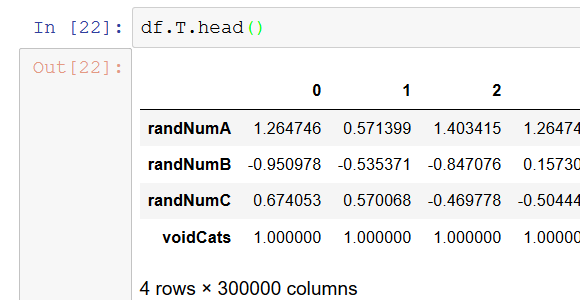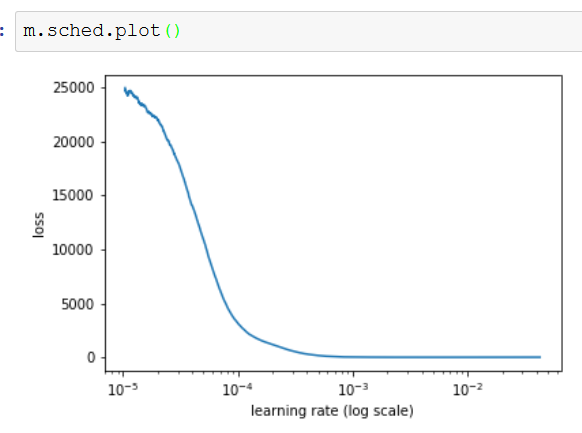# Problem prediction columnar data

(Anders) #1

TL:DR How do I get predictions from ColumnarData?

After course DL1:4 (Rossmann structured data) I try to train a NN on some synthetic data. 300.000 rows of random variables between 0 and 100.
E.g.
A, B, C
50, 2, 81

My dependend variable is A + B + C. So I expect the NN to roughly predict Y = A+B+C.

Then I found out that `ColumnarModelData.from_data_frame` MUST have categorical data, so I added a dummy column ‘voidCats’ and set the column to string ‘None’.
Then I set the types, normalize, proc_df(), etc. and this is my dataframe:This is my learning rateBut when I try to do a prediction I run into trouble:

test_ds = ColumnarDataset.from_data_frame(df[:1],cat_flds=‘voidCats’)
preds = m.predict_dl(test_dl)

The first line fails with:

~/fastai/courses/dl1/fastai/column_data.py in (.0)
39 @classmethod
40 def from_data_frames(cls, df_cat, df_cont, y=None, is_reg=True, is_multi=False):
—> 41 cat_cols = [c.values for n,c in df_cat.items()]
42 cont_cols = [c.values for n,c in df_cont.items()]
43 return cls(cat_cols, cont_cols, y, is_reg, is_multi)

AttributeError: ‘int’ object has no attribute ‘values’

Did I mess something up the dummy-category?

(Anders) #2

Note I do realize that I can’t just copy-paste get_next() and use it.

I’m digging around to solve this and tried to copy the solution from dl1/lesson6-rnn. It looks like this:

def get_next(inp):
idxs = T(np.array([char_indices[c] for c in inp]))
p = m(*VV(idxs))
i = np.argmax(to_np§)
return chars[i]

Then I realize that the modle from lesson 3 is different! The rossman model is a “StructuredLearner” and you cannot pass variables to it. It is also trained in a different way. `m.fit` rather than `fit(m, ...)`.

So if I cannot pass variables to StructuredLearner, how do I make predictions with it?